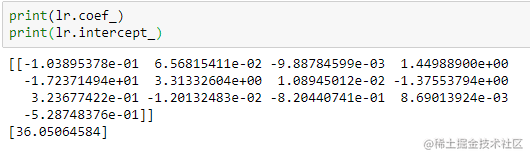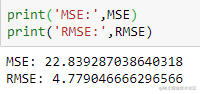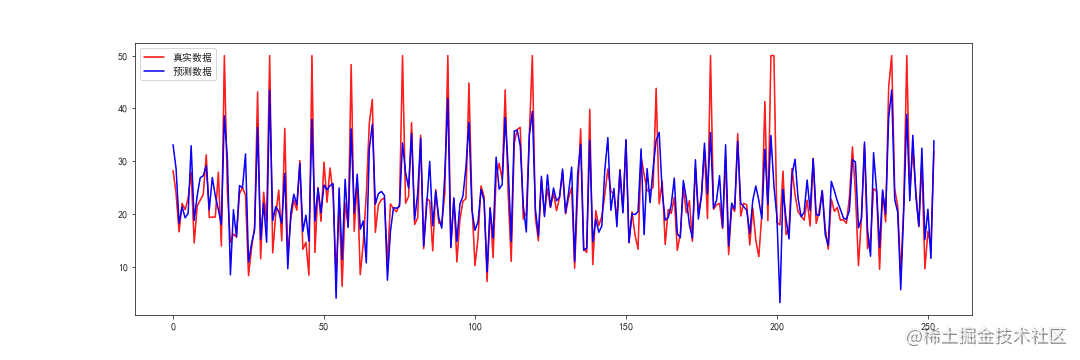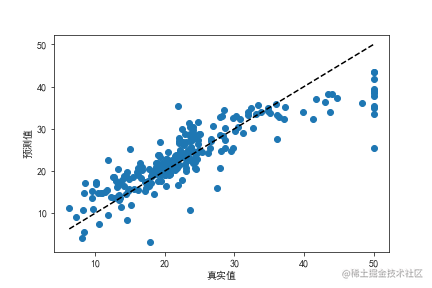sklearn：多元线性回归，模型训练/评估/调优1/数据集的导入以及自变量和因变量的选取

import pandas as pd
import matplotlib.pyplot as plt
import matplotlib as mpl
from sklearn.linear_model import LinearRegression
from sklearn.model_selection import cross_val_predict, train_test_split
from sklearn import datasets
%matplotlib inline
mpl.rcParams['font.family'] = ['sans-serif']
mpl.rcParams['font.sans-serif'] = ['SimHei']
mpl.rcParams['axes.unicode_minus']=False

# 构建一个dataframe数据对象,data.data是自变量数据
x_df = pd.DataFrame(data=data.data,columns=data.feature_names)

# 构建一个dataframe数据对象，data.target是因变量数据
y_df = pd.DataFrame(data=data.target,columns=['MEDV'])
x = x_df
y = y_df

2/划分训练集和测试集

x_train,x_test,y_train,y_test = train_test_split(x,y, test_size=0.5, random_state=1)
# test_size=0.5就是把原始数据的一半作为测试集
print(x_train.shape)  # (253,13)  253行，13列
print(x_test.shape)

3/模型的训练

#划分好训练集和测试集后，就是对模型进行训练了，然后我们输出截距和权重系数，看下模型
lr = LinearRegression()
lr.fit(x_train, y_train)  # 把训练集的自变量，因变量添加到函数fit()中，进行训练
print(lr.coef_)  # lr.coef_得到的是每个自变量的权重系数
print(lr.intercept_)  # lr.intercept_得到的是截距4.模型的评估

y_pred = lr.predict(x_test)
from sklearn import metrics
MSE = metrics.mean_squared_error(y_test, y_pred)
RMSE = np.sqrt(metrics.mean_squared_error(y_test, y_pred))

print('MSE:',MSE)
print('RMSE:',RMSE)plt.figure(figsize=(15,5))
plt.plot(range(len(y_test)), y_test, 'r', label='测试数据')
plt.plot(range(len(y_test)), y_pred, 'b', label='预测数据')
plt.legend()plt.scatter(y_test, y_pred)
plt.plot([y_test.min(),y_test.max()], [y_test.min(),y_test.max()], 'k--')
plt.xlabel('真实值')
plt.ylabel('预测值')5.模型调优

# 去除MEDV=50的异常值
drop_index = y[y['MEDV']==50].index.values
x = X.drop(drop_index)
y = y.drop(drop_index)
# 这里划分训练集和测试集的参数random_state都是1，表示随机分配的数据是同一组
x_train, x_test, y_train, y_test = train_test_split(x, y, test_size=0.25, random_state=1)  # test_size=0.25,说明训练样本多了
# 对训练集进行训练
lr = LinearRegression()
lr.fit(x_train, y_train)
# 对测试集进行预测
y_pred = lr.predict(x_test)
RMSE = np.sqrt(metrics.mean_squared_error(y_test, y_pred))
print('RMSE:',RMSE)  # 得到RMSE指标是3.573

1. 增加了训练集的数量，提高训练准确度；
2. 去除异常值，提高准确度。

6/总结：

LinearRegression本身的参数只有四个，大多数情况下也不需要进行参数调整，默认的就OK。

SSE(和方差、误差平方和)：The sum of squares due to error
MSE(均方差、方差)：Mean squared error
RMSE(均方根、标准差)：Root mean squared error
R-square(确定系数)：Coefficient of determination

该统计参数计算的是拟合数据和原始数据对应点的误差的平方和，计算公式如下：
均方根值（RMS）+ <wbr>均方根误差（RMSE）
SSE越接近于0，说明模型选择和拟合更好，数据预测也越成功。接下来的MSE和RMSE因为和SSE是同出一宗，所以效果一样

该统计参数是预测数据和原始数据对应点误差的平方和的均值，也就是SSE/n，和SSE没有太大的区别，计算公式如下：
均方根值（RMS）+ <wbr>均方根误差（RMSE）

该统计参数，也叫回归系统的拟合标准差，是MSE的平方根，就算公式如下
均方根值（RMS）+ <wbr>均方根误差（RMSE）

在讲确定系数之前，我们需要介绍另外两个参数SSR和SST，因为确定系数就是由它们两个决定的
(1)SSR：Sum of squares of the regression，即预测数据与原始数据均值之差的平方和，公式如下
均方根值（RMS）+ <wbr>均方根误差（RMSE）

(2)SST：Total sum of squares，即原始数据和均值之差的平方和，公式如下
均方根值（RMS）+ <wbr>均方根误差（RMSE）

细心的网友会发现，SST=SSE+SSR，呵呵只是一个有趣的问题。而我们的“确定系数”是定义为SSR和SST的比值，故
均方根值（RMS）+ <wbr>均方根误差（RMSE）

其实“确定系数”是通过数据的变化来表征一个拟合的好坏。由上面的表达式可以知道“确定系数”的正常取值范围为[0，1]，越接近1，表明方程的变量对y的解释能力越强，这个模型对数据拟合的也较好.